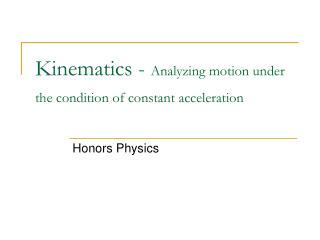DownloadDownload PresentationKinematics - Analyzing motion under the condition of constant acceleration

# Kinematics - Analyzing motion under the condition of constant acceleration

Télécharger la présentation## Kinematics - Analyzing motion under the condition of constant acceleration

- - - - - - - - - - - - - - - - - - - - - - - - - - - E N D - - - - - - - - - - - - - - - - - - - - - - - - - - -
##### Presentation Transcript

1. Kinematics - Analyzing motion under the condition of constant acceleration Honors Physics

2. Kinematic Symbols

3. Kinematic #1

4. Kinematic #1 Example:A boat moves slowly out of a marina (so as to not leave a wake) with a speed of 1.50 m/s. As soon as it passes the breakwater, leaving the marina, it throttles up and accelerates at 2.40 m/s/s. a) How fast is the boat moving after accelerating for 5 seconds? 13. 5 m/s

5. Kinematic #2 b) How far did the boat travel during that time? 37.5 m

6. Does all this make sense? 13.5 m/s 1.5 m/s Total displacement = 7.50 + 30 = 37.5 m = Total AREA under the line.

7. Kinematic #3 Example:You are driving through town at 12 m/s when suddenly a ball rolls out in front of your car. You apply the brakes and begin decelerating at 3.5 m/s/s. How far do you travel before coming to a complete stop? 20.57 m

8. Common Problems Students Have I don’t know which equation to choose!!!

9. Kinematics for the VERTICAL Direction All 3 kinematics can be used to analyze one dimensional motion in either the X direction OR the y direction.

10. Examples A pitcher throws a fastball with a velocity of 43.5 m/s. It is determined that during the windup and delivery the ball covers a displacement of 2.5 meters. This is from the point behind the body to the point of release. Calculate the acceleration during his throwing motion. Which variable is NOT given and NOT asked for? TIME

11. Examples How long does it take a car at rest to cross a 35.0 m intersection after the light turns green, if the acceleration of the car is a constant 2.00 m/s/s? Which variable is NOT given and NOT asked for? Final Velocity

12. Examples A car accelerates from 12.5 m/s to 25 m/s in 6.0 seconds. What was the acceleration? Which variable is NOT given and NOT asked for? DISPLACEMENT

13. Examples A stone is dropped from the top of a cliff. It is observed to hit the ground 5.78 s later. How high is the cliff? Which variable is NOT given and NOT asked for? Final Velocity